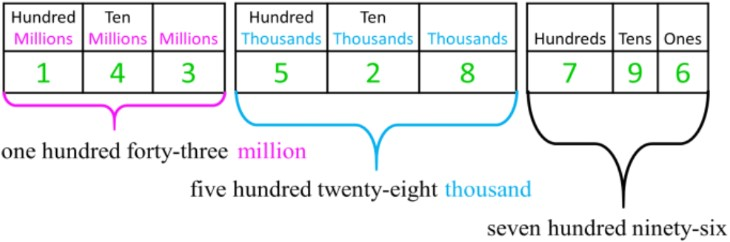# Whole number place values up to millions#### Everything You Need in One Place

Homework problems? Exam preparation? Trying to grasp a concept or just brushing up the basics? Our extensive help & practice library have got you covered.#### Learn and Practice With Ease

Our proven video lessons ease you through problems quickly, and you get tonnes of friendly practice on questions that trip students up on tests and finals.#### Instant and Unlimited Help

Our personalized learning platform enables you to instantly find the exact walkthrough to your specific type of question. Activate unlimited help now!

0/4
##### Intros
###### Lessons
1. Introduction to Whole Number Place Values up to Millions:
2. Naming and understanding whole number place values up to the millions group
3. Write large whole numbers in standard form
4. Writing large whole numbers in expanded form
5. Writing large whole numbers in written form
0/15
##### Examples
###### Lessons
1. Writing place value names up to millions
Write the place value name for the underlined digit.
1. 6 706
2. 235,914
3. 8,706,539
4. 941 285 734
2. Writing values of place value digits up to millions
Write the value of the 7 in the number.
1. 5,719
2. 372 048
3. 764,158,609
3. Place values up to millions in expanded form
Fill in each box with the value of each digit and then write in expanded form (with both multiplication and addition).
1. 12,914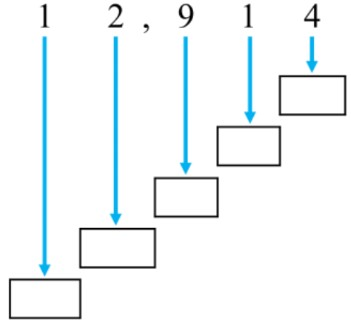2. 360,578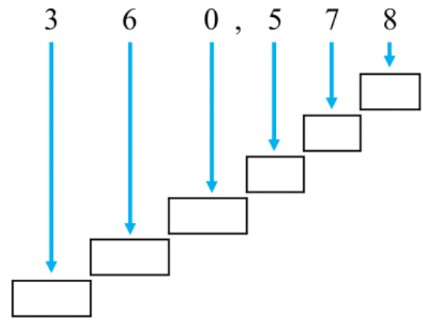3. 54 196 784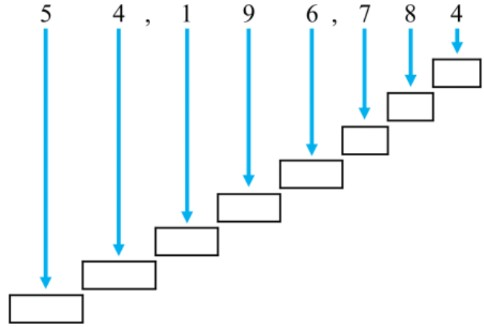4. Place values up to millions in standard form
Turn the written number into a number in standard form.
1. four hundred sixty-two thousand eight hundred thirteen
2. fifty million six hundred ninety-seven thousand three hundred eighty-four
3. two hundred eighty-six million one hundred forty-five thousand three hundred seventy
5. Place values up to millions in written form
Write each number with words (in written form).
1. The planet Jupiter has a distance of 778,330,000 km from the sun.
2. The circumference of Earth is about 40,075,017 m.
0%
##### Practice
###### Topic Notes

In this lesson, we will learn:

• The names and values of place values to the millions
• How to write large whole numbers up to millions in standard form, expanded form, and written form

Notes:

• Place value names can be memorized by looking at the pattern of every three place values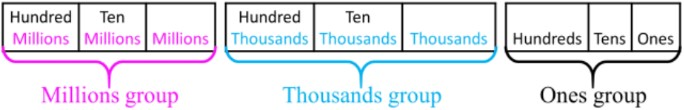• The standard form of large whole numbers should have commas (,) or spaces between every three place values (every 3 digits, starting from the ones place)
• Ex. 43528796 should be written as: 43 528 796 OR 43,528,796 for clarity
• The value of each digit of each place value is related to powers of 10: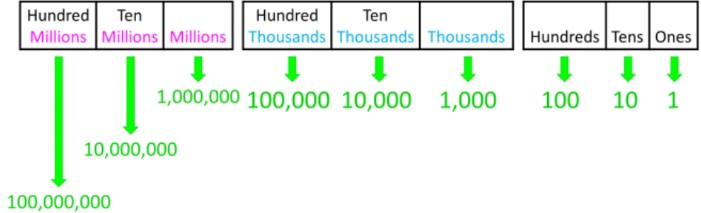• The expanded form of a number shows all the values of each digit as a sum with either addition only OR both addition and multiplication.
• Ex. 126 in expanded form is: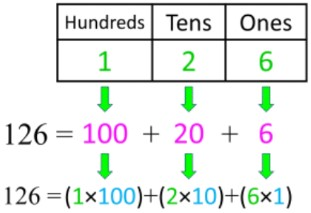• The written form of a number uses words to name the whole number
• Name each group of 3 digits, then write the name of the group ("million" or "thousand")
• Ex. 143,528,796 in written form is: